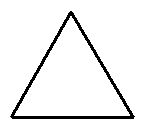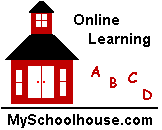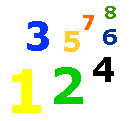# Squares, Circles, Cylinders, & Spheres

Shapes & Solids Lesson - Solids Worksheet - Shapes Worksheets - My Schoolhouse - Online Learning

Math that uses triangles, rectangles, squares, and circles is called geometry.  Look at the shapes below to learn about  geometry.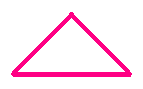Triangle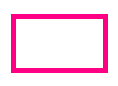Rectangle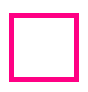Square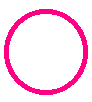CircleA triangle has three sides. A rectangle has four sides. A square has four sides of  equal length. A circle is round and has a center point.

Below are solid shapes.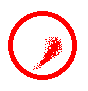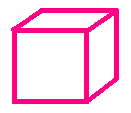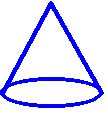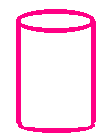Sphere Cube Cone Cylinder

Name the shapes below.